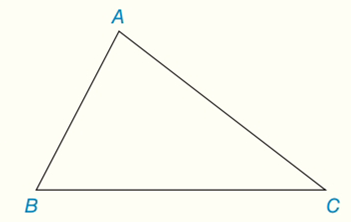Chapter 7.CT, Problem 6CTElementary Geometry For College St...

7th Edition
Alexander + 2 others
ISBN: 9781337614085

Solutions

Chapter
SectionElementary Geometry For College St...

7th Edition
Alexander + 2 others
ISBN: 9781337614085
Textbook Problem

For a given triangle (such as △ A B C ), what word describes the point of concurrency fora) the three angle bisectors?b) the three medians?Exercises 6, 7

To determine

(a)

To describe:

The point of concurrency for the three angle bisectors for a given triangle (such as ABC).

Explanation

The incenter is the center of the triangle's incircle, the largest circle that will fit inside the triangle and touch all three sides.

One of several centers the triangle can have, the incenter is the point where the angle bisectors intersect. The incenter is also the center of the triangle's incircle- the largest circle that will fit inside the triangle.

Calculation:

The point where the three internal angle bisectors of a triangle are concurrent is called the incenter of the triangle.

Suppose we bisect the internal angles of ABC

To determine

(b)

To describe:

The point of concurrency for the three medians for a given triangle (such as ABC).

Still sussing out bartleby?

Check out a sample textbook solution.

See a sample solution

The Solution to Your Study Problems

Bartleby provides explanations to thousands of textbook problems written by our experts, many with advanced degrees!

Get Started

Subtract and check: 98,40572,397

Elementary Technical Mathematics

Find the slope of the line through P and Q. 10. P(1, 4), Q(6, 0)

Single Variable Calculus: Early Transcendentals, Volume I

In Exercises 1124, find the indicated limits, if they exist. 21. limxx2x21

Applied Calculus for the Managerial, Life, and Social Sciences: A Brief Approach

For , which vector, a, b, c, or d, best represents F(1, 1)? a b c d

Study Guide for Stewart's Multivariable Calculus, 8th

The length of the are r = e for 0 is given by: a) 0ed b) 02e2d c) 02ed d) 02ed

Study Guide for Stewart's Single Variable Calculus: Early Transcendentals, 8th